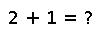# randsubseq

randsubseq(A, p) -> Vector

Return a vector consisting of a random subsequence of the given array `A`, where each element of `A` is included (in order) with independent probability `p`. (Complexity is linear in `p*length(A)`, so this function is efficient even if `p` is small and `A` is large.) Technically, this process is known as "Bernoulli sampling" of `A`.

## Examples

Checking you are not a robot: